# The Blipos Clock

Recall the Software Development Process from Chapter 2. We will be using relevant elements from this set of recommended activities. The complete source code is provided in Listing 6.8 and will be referenced throughout the discussion.

Software Specification:

The program must show the user how time keeping is performed on Blipos. We will not try to create a fully autonomic clock, merely construct a "clock" that can be set initially through appropriate commands and then adjusted by increments and decrements of 1 second or 50 seconds. After each adjustment, the new time of the clock will be shown onscreen in the form of: Sec: sss Min: mmmmm, where sss is an integer between 0 and 255 and mmmmm is an integer between 32768 and +32767. The user can make any adjustments one after the other by entering different keys. Entering a T (for terminate) will stop the program.

Software Design:

Segregating each subsystem into objects (modules).

We can identify one obvious object in the software specification a Blipos clock (class name BliposClock) representing a clock that follows the same rules for time keeping as a clock on Blipos. This clock will encapsulate the instance variables seconds and minutes, which represent the time of the clock.

Identify the methods in each module.

The BliposClock has several public methods that allow it to be manipulated in suitable ways. According to the specification, it should be possible to

• Set the clock.

• Move it one second forward, one second backward, 50 seconds forward, and 50 seconds backward.

• Ask it to show its current time.

The Main method is the control center of the program. It creates an instance of the BliposClock class and repeatedly allows the user to give commands to this object, adjusting its time, until the user enters a T, which terminates the loop and the program. Every time the user has changed the time, the clock object is asked to display its current time.

Internal Method Design:

We will focus on solving two important problems to get the program ticking:

• Problem 1 We attempt to utilize the overflow/underflow mechanism to implement the behavior of seconds. Just as the seconds of a digital watch "overflow" when the seconds counter reaches 59 and returns to 00, our watch will overflow when it reaches 255 seconds and return to 0.

The following problem must be solved to write any of the methods in the program that increment or decrement the seconds instance variable of a BliposClock object.

Every time the seconds instance variable overflows, it must trigger the minutes instance variable to be incremented by 1, just like our watch increments the minutes display whenever the seconds counter "overflows" at 59 seconds. Conversely, minutes must be decremented by 1 every time seconds underflows. The important question is how do we detect when the overflow and underflow is taking place so we can adjust the minutes variable correctly?

• Problem 2 The program must somehow repeat itself in a loop until the user enters the letter T. How is this implemented?

The following is the solution to Problem 1, detecting an overflow.

When the seconds variable is incremented without an accompanying overflow, the following is true: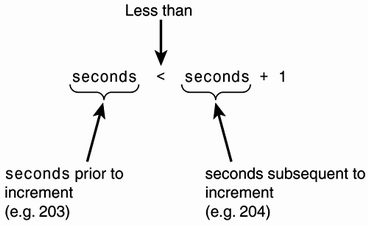In other words, prior to the increment, seconds is less than seconds subsequent to the increment if no overflow takes place.

But if an overflow takes place, this no longer holds; instead, the following statement becomes true:Thus, by "remembering" the value of seconds prior to its increment, we can use this piece of memory (here conveniently called originalSeconds) to compare to the new value of seconds and determine whether an overflow has taken place.

The algorithm used to check for overflow and adjust minutes is shown in Listing 6.6 using pseudocode.

##### Listing 6.6 Pseudocode: Overflow Detection Algorithm
`Algorithm inside OneForward method which increments seconds by one. 01: Set the variable originalSeconds equal to seconds 02: Increment seconds by 1 03: If originalSeconds is greater than seconds then perform line 4 else jump over line 4to line 5. 04:          Increment minutes by 1 (seconds have just overflown) 05: End `

The same logic applies to detection of underflow in the OneBackward method. Have a look at the OneForward and OneBackward methods in lines 24 32 and 34 42 of Listing 6.8 and compare with the pseudocode. To remember the original value of seconds before it is incremented, we use the local variable originalSeconds.

Local Variables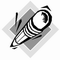Because originalSeconds is declared within a method body, we call it a local variable. A local variable is confined to the method body where it is declared. Consequently, originalSeconds declared in OneBackward is entirely different from originalSeconds in OneForward. They only share the same name.

The FastForward and FastBackward methods apply the same overall logic as OneBackward and OneForward.

The method of applying intermediate values, such as originalSeconds, to solve a programmatic problem is commonly used in programming.

The solution for Problem 2, creating a loop for user input, can be expressed by pseudocode, as presented in Listing 6.7.

##### Listing 6.7 Pseudocode: A Loop for User Input
`01: Begin loop 02:      Pause program and wait for user input. Read the command given by the user andstore it in a variable, here called command. 03:      If command is an "F" then move the clock one second forward. 04:      If command is a "B" then move the clock one second backwards. 05:      If command is an "A" then move the clock forward by 50 seconds. 06:      If command is a "D" then move the clock backward by 50 seconds. 07:      Show the time of the clock 08: Repeat loop if command is not equal to "T" (for Terminate), by starting at firststatement after Begin in line 01. If command is equal to "T" then terminate the loop. `

Notice how lines 2 7 are repeated as long as the command variable does not hold the value T.

Compare this algorithm with lines 97 109 of Listing 6.8. To implement the loop in Listing 6.7, a do-while loop is applied. Line 1 of the pseudocode corresponds to do of line 97, and line 8 corresponds to line 109 of Listing 6.8. Notice that != in line 109 means "is not equal to." The do-while loop will repeat lines 99 108 until the condition inside the parenthesis after while in line 109 is false. This occurs when the user has given the T command.

Take a moment to look through the source code in Listing 6.8. Even though it contains a few new C# elements, most of it should be familiar to you by now. All the new features are briefly explained shortly.

To illustrate overflow and underflow in the sample output presented after the source code, I set the clock to 253 seconds and 32,767 minutes. By entering f, the clock is moved forward by one second to 254 seconds and 32,767 minutes. However, when the seconds instance variable of the clock reaches 255 with an attempt to increment it, an overflow takes place and its value returns to the beginning of byte's range (0). The overflow is detected by the program, triggering the minutes instance variable of myClock to be incremented by 1. But minutes is of type short, so this variable also experiences an overflow, this time resulting in new value of 32,767. By entering f again, we simply increment seconds by one without affecting minutes. If we enter b twice, the clock is adjusted backwards, triggering an underflow and causing seconds to return from 255 to 0.

Finally, the sample output demonstrates the effect of entering a and d, adjusting the clock 50 seconds forward and 50 seconds backward, respectively.

##### Listing 6.8 Source Code for BliposClock.cs
` 01: using System;  02:  03: /*  04:  * The Blipos clock has 256 seconds in a minute and  05:  * 65536 minutes in a day. The night lasts for  06:  * 32768 minutes and is represented by negative minute values.  07:  * The daylight lasts 32767 minutes represented by positive  08:  * values. When minutes equals 0 it is neither day nor night.  09:  * The clock can tick forwards, backwards, be adjusted,  10:  * move fast forwards and fast backwards  11:  */  12:  13: class BliposClock  14: {  15:     private byte seconds;  16:     private short minutes;  17:  18:     public BliposClock()  19:     {  20:         seconds = 0;  21:         minutes = 0;  22:     }  23:   24:     public void OneForward()  25:     {  26:         byte originalSeconds = seconds;  27:  28:         seconds++;  29:         if(originalSeconds > seconds)  30:              // Overflow of seconds variable  31:             minutes++;  32:     }  33:  34:     public void OneBackward()  35:     {  36:         byte originalSeconds = seconds;  37:  38:         seconds--;  39:         if (originalSeconds < seconds)  40:              // Underflow of seconds variable  41:             minutes--;  42:     }  43:  44:     public void FastForward()  45:     {  46:         byte originalSeconds = seconds;  47:  48:         seconds = (byte)(seconds + 50);  49:         if (originalSeconds > seconds)  50:              // Overflow of seconds variable  51:             minutes++;  52:     }  53:   54:     public void FastBackward()  55:     {  56:         byte originalSeconds = seconds;  57:  58:         seconds = (byte)(seconds - 50);  59:         if (originalSeconds < seconds)  60:              // Underflow of seconds variable  61:             minutes ;  62:     }  63:  64:     public void SetSeconds(byte sec)  65:     {  66:         seconds = sec;  67:     }  68:  69:     public void SetMinutes(short min)  70:     {  71:         minutes = min;  72:     }  73:  74:     public void ShowTime()  75:     {  76:         Console.WriteLine("Sec: " + seconds + " Min: " + minutes);  77:     }  78: }  79:  80: class RunBliposClock  81: {  82:     public static void Main()  83:     {  84:         string command;  85:   86:         Console.WriteLine("Welcome to the Blipos Clock. " +  87:             "256 seconds per minute " +  88:             "65536 minutes per day");  89:         BliposClock myClock = new BliposClock();  90:         Console.WriteLine("Please set the clock");  91:         Console.Write("Enter Seconds: ");  92:         myClock.SetSeconds(Convert.ToByte(Console.ReadLine());  93:         Console.Write("Enter minutes: ");  94:         myClock.SetMinutes(Convert.ToInt16(Console.ReadLine());  95:         Console.WriteLine("Enter orward ackward " +  96:             "dd fifty educt fifty (T)erminate");  97:         do  98:         {  99:             command = Console.ReadLine().ToUpper(); 100:             if (command == "F") 101:                 myClock.OneForward(); 102:             if (command == "B") 103:                 myClock.OneBackward(); 104:             if(command == "A") 105:                 myClock.FastForward(); 106:             if(command == "D") 107:                 myClock.FastBackward(); 108:             myClock.ShowTime(); 109:         }  while (command != "T"); 110:         Console.WriteLine("Thank you for using the Blipos Clock"); 111:     } 112: } Welcome to the Blipos Clock. 256 seconds per minute 65536 minutes per day Please set the clock Enter Seconds: 253<enter> Enter minutes: 32767<enter> Enter orward ackward dd fifty educt fifty (T)erminate f<enter> Sec: 254 Min: 32767 f<enter> Sec: 255 Min: 32767 f<enter>         (Overflowing here. This comment is not part of output). Sec: 0 Min: -32768 f<enter> Sec: 1 Min: -32768 b<enter> Sec: 0 Min: -32768 b<enter>           (Underflow is taking place here) Sec: 255 Min: 32767 a<enter>           (Overflow is taking place here) Sec: 49 Min: -32768 d<enter>           (Underflow is taking place here) Sec: 255 Min: 32767 t<enter> Thank you for using the Blipos Clock `

Lines 18 22 constitute the constructor of the BliposClock class. Whenever a new object of this class is created, this constructor will be called and will initialize the instance variables seconds and minutes to 0.

The method header of a method called OneForward is in line 24. It increments the seconds instance variable of BliposClock by 1 and increments minutes by 1 if seconds overflows.

Line 26 declares a local variable called originalSeconds of type byte and assigns it the value of the instance variable seconds.

Line 28 uses the increment operator ++ to increase the value of seconds by 1.

Line 29 is the first line of an if statement. It applies the comparison operator > (greater than) to determine whether originalSeconds is greater than second.

If originalSeconds is greater than seconds, line 31 executes and increments minutes by 1.

Line 34 is the method header of OneBackward, which, when called, will decrease the seconds instance variable by 1 and decrement minutes by 1 if seconds underflows.

Line 38 decrements the instance variable seconds by 1, using the decrement operator --.

Lines 39 41 use the comparison operator < (less than) to determine whether originalSeconds is less than seconds. If this is the case, decrement minutes by 1 with the decrement operator.

In lines 44 52, the FastForward method increases seconds by 50 and increments minutes by 1 if seconds experiences any overflow during this operation.

In line 48, the expression (seconds + 50) is of type int and cannot be assigned to seconds of type byte. Consequently, we use the type cast (byte) in (byte)(seconds + 50), which produces the value (seconds + 50) of type byte. Type casts are discussed in more detail in a later section of this chapter titled "Explicit Type Conversions."

Line 89 creates an object of class BliposClock and assigns the reference of this object to myClock.

The code block surrounded by the braces in lines 98 and 109 (lines 99 108) is repeated as long as (command != "T") is true due to the do-while loop. != is a comparison operator called "not equal to," so as long as command is not equal to "T", the code block of lines 99 108 will be repeated. As soon as the user types a T, the loop is terminated.

Lines 100 107 consist of several if statements to determine which command the user has given. For example, entering a D (or d) will move the clock FastBackward.

Do Not Rely on Overflow/Underflow to Implement Logic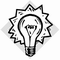The source code in Listing 6.8 has been created as a teaching tool to illustrate overflow and underflow through this mechanism's resemblance to the workings of a clock. However, it is not generally recommendable to rely on overflow and underflow in your source code to implement the logic of a program. C# might well one day change the way it handles overflow/underflow or perhaps change the ranges of the involved types and, consequently, introduce bugs into your programs.

#### Working with Integer Types

The next two sections provide a few useful hints when you apply integer types in your programs.

##### Preventing Overflow and Underflow Exceptions

Even though you can always fall back on the compiler to create checks for overflow and underflow during the design and testing of your program, the best way to avoid these kinds of exceptions is to think through each of the expressions that involve integers in your program.

Try to figure out the largest and smallest value each expression of type integer can possibly reach. For example, if you have an expression such as (pricePerKilo * amountOfKilos), you will have to work out the probable minimum and maximum values of the two involved variables. By calculating max pricePerKilo with max amountOfKilos, you can find the probable maximum value for the whole expression. Similar logic is applied to find the minimum amount of the expression. In these calculations, you will have to consider future possible values of the involved variables. So even though pricePerKilo has fluctuated between 20 and 100 for the last 10 years, that doesn't necessarily mean it cannot suddenly reach 200 the next year.

It is important to check every sub-expression of a larger expression, not just the overall end result. Consider the simple source code in Listing 6.9. What is the output? An initial guess might be 1,000,000, but this is not the case here. Why? First, all the sub-parts of the expression in line 9 are of type int. Second, to calculate this expression, the sub-expression 1000000 * 1000000 will have to be calculated first. This is where our problems start. The result of this calculation is, in reality, 1,000,000,000,000, which is larger than the maximum possible int value of 2147483647, so the initial multiplication causes an overflow with the result of 727379968. The program finally divides this number by 1,000,000, producing the unexpected and incorrect result of 727.

##### Listing 6.9 Source Code for OverflowingSubExpression.cs
`01: using System; 02: 03: class OverflowingSubExpression 04: { 05:     public static void Main() 06:     { 07:         int result; 08: 09:         result = 1000000 * 1000000 / 1000000; 10:         Console.WriteLine("Result of calculation: " + result); 11:     } 12: } `

Because of these incorrect results the compiler generates the following error message if you attempt to compile Listing 6.9:

` OverflowingSubExpression.cs(9.18): error CS0220: The operation overflows at  compile time in checked mode `

Only literals are involved in line 9 of Listing 6.9. Because literals stay unchanged during the execution of a program, the compiler can identify the problem in line 9 at compile time.

##### Disappearing Fractions

What is the result of the expression (4 / 10) x 10? Under normal circumstances, it would be 4, but calculating this expression using integers in the source code returns a 0. The result of the sub-expression (4 / 10) is 0.4, which is represented as a 0 when using integers so the fraction .4 has been discarded. Multiplying 0 by 10 results in 0.

To prevent this type of error, you can attempt to rearrange the expression. In this case, you could write the expression as (4 * 10) / 10. If a reordering turns out to be impossible, you should probably resort to the floating-point or decimal types, which are designed to handle numbers with fractions.

#### Floating-Point Types

Floating-point types differ from integer types in many important ways. In contrast to variables of type integer, floating-point variables let you store numbers with a fraction, such as 2.99 (the price of the "Mock Chicken Salad" I happen to be eating right now) or a number like 3.1415926535897931, which is an approximation of the mathematical constant Pi (p).

Floating-point numbers also enable you to represent a much wider range of values than the most expansive integer type long. Even the number 9,223,372,036,854,775,807 (the maximum in the range of long) pales compared to the largest possible floating-point number, which is equivalent to 17 with 307 zeros behind it. Similarly, floating-point numbers also let you store extremely large negative numbers.

##### Two Floating-Point Notations Used in C#

Floating-point numbers are often used when representing very large or small numbers. When writing numbers of such magnitude, it is often convenient to use a notation called scientific notation, also called e-notation or floating-point notation.

This notation expresses the number 756,000,000,000,000 as shown in Figure 6.14, but this notation cannot be used in C# source code.

##### Figure 6.14. Scientific notation.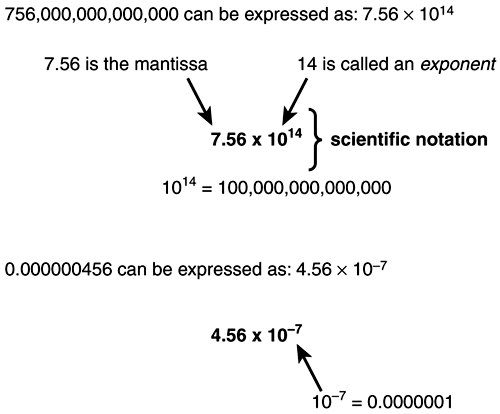C# allows you to write floating-point numbers using two different notations. You can use the form we are familiar with from everyday life with a decimal point followed by digits (for example 134.87 and 0.0000345), or you can use the scientific notation shown here. However, because it is difficult to write exponents with the keyboard (it took me a while before I knew how to type the 7 in 107 using MS Word), the multiplication sign and the 10 has been removed and replaced with an E (or e) with the exponent written after the E. For example, the number 0.000000456 (equal to 4.56 x 10-7) can be written in C# as +4.56E-7. Figure 6.15 looks closer at each part of this notation form.

##### Figure 6.15. A closer look at scientific notation.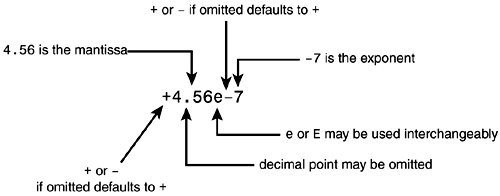The following are a few floating-point numbers written as they look in a C# program using our familiar everyday notation:

` 56.78      // floating-point every day notation 0.645      // floating-point 7.0        // also floating-point. `

Notice that even though the fraction in the last line is 0, the decimal point still triggers the compiler to treat 7.0 as a floating-point number.

The following are a few floating-point numbers in C# using e-notation:

` 456E-7      //same as 4.56e-7 8e3         //same as +8.0e+3 still floating-point. -8.45e8     //a negative value 1.49e8      //distance between the earth and the sun 8.88e11     //distance from earth to planet Blipos in miles. 9.0e-28     //the mass of an electron in grams `
##### The Floating (Decimal) Point

You might wonder why these types are called floating-point types. Well actually the (decimal) point in a floating-point number is "floating," meaning that it can be moved to the right or left in an otherwise unchanging sequence of digits. Let's look at this mechanism a bit closer.

You can think of a floating-point number as consisting of two parts, a base value and a scaling factor that moves the decimal point of the base value to the right or the left. Consider a base value of 0.871254; a scaling factor of 100 (102) would move the decimal point two places to the right, resulting in the number 87.1254. A scaling factor of 10000 (104) produces the number 8712.54 by moving the decimal point four places to the right. Conversely, it would be possible to move the decimal point to the left and create a smaller fraction closer to 0. For example, with a scaling factor of 0.001 (10-3), we would move the decimal point three places to the left, producing 0.000871254.

NoteEven though we can scale the base value 0.871254 up or down, the digits and their sequence (871254) remain unchanged.

Interpreting the E-Notation4.56E+7 means "Take the mantissa 4.56 and move the decimal point 7 places to the right; insert zeros as placeholders when the decimal point moves away from the digit farthest to the right." 4.56E-7 means "Take the mantissa 4.56 and move the decimal point 7 places to the left; insert zeros as placeholders when the decimal point moves away from the digit farthest to the left."

NoteThe maximum number of digits that can be represented by the base value varies between the two floating-point types found in C# and so is an important factor for determining the range and the accuracy of each type.

##### The Two Floating-Point Types

C# has two floating-point types, float and double. Two main attributes distinguish these two types the number of significant digits they can represent (related to the base value concept already discussed) and the range of the exponents (related to the scaling factor concept).

float and double in a NutshellVariables of the float type can hold values from 3.4E38 to 3.4E38. They can get as close to zero (without holding zero itself) as 1.5e 45 or 1.5e 45. Values are represented with approximately 7 significant digits. A float occupies 32 bits (4 bytes) of memory. A variable of the double type can hold values from 1.7E308 to 1.7E308. It can get as close to zero (without holding zero itself) as 5.0E 324 or 5.0E 324. Values are represented with 15 16 significant digits. A double occupies 64 bits (8 bytes) of memory. The definitions of and operations on C#'s floating-point types follow the IEEE 754 specifications, a commonly used standard. More details are available at IEEE's Web site at http://www.ieee.org.

The differing number of significant digits between the two types influence their accuracy. Thus, to write a number that can accurately be represented by the double type, it must contain less than approximately 17 significant digits. For example, the number 123,456,789,012,345 consists of 15 significant digits. If we tried to squeeze (explicitly convert) this number into a variable of type float, it would only be able to represent it as 123,456,700,000,000 containing only 7 significant digits. The remaining eight zeros are merely used as placeholders. This is illustrated in Figure 6.16.

##### Figure 6.16. "Squeezing" a double into a float.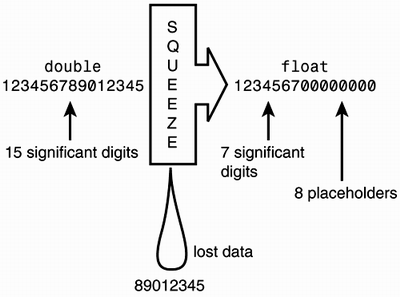NoteThe ranges and number of significant digits for float and double, shown in the "Float and Double in a Nutshell" Note, are only approximations. When I tried to "squeeze" 123,456,789,012,345 into a float on my computer, it represented the number as 123,456,788,000,000 deviating from 123,456,700,000,000 previously stated.

Recall how an overflowing/underflowing integer value could generate an exception and then cause the program to terminate. In contrast, operations on floating-point values never produce exceptions during abnormal situations; instead, one of the following results is produced, depending on the operation performed:

• Positive zero (+0) or negative zero (-0)

• Positive infinity () or negative infinity ()

• NaN (Not a Number)

Each of these results, and the situations triggering them, are summarized in the following Note. Please note that the information provided here is of a more technical nature and is not required to understand any parts introduced later in the book.

Abnormal Floating-Point Operations and Their Results (Optional)If an attempt is made to assign a value x to a variable of type float, where x can be defined as 1.5E 45 > x > 1.5E-45 and x0 (in other words, if x is very close to zero, without being zero), x cannot be represented properly by the float variable. The result of the operation is a positive zero (if x is positive) or a negative zero (if x is negative). If an attempt is made to assign a value x to a variable of type double, where x can be defined as 5.0E 324 > x > 5.0E 324 and x0 (in other words, if x is very close to zero, without being zero), x cannot be represented properly by the double variable. The result of the operation is a positive zero (if x is positive) or a negative zero (if x is negative). If an attempt is made to assign a value x to a variable of type float, where x can be defined as 3.4E38 > x or x > 3.4E38 (in other words, if the magnitude of x is too large either in a negative direction or in a positive direction), the result of this operation becomes negative infinity (denoted - Infinity in output from the C# program) or positive infinity (denoted Infinity in output from the C# program). If an attempt is made to assign a value x to a variable of type double, where x can be defined as 1.7E308 > x or x > 1.7E308 (in other words, if the magnitude of x is too large either in a negative direction or in a positive direction), the result of this operation becomes negative infinity (denoted - Infinity in output from the C# program) or positive infinity (denoted Infinity in output from the C# program) If an attempt is made to perform an invalid operation on a floating-point type, the result is NaN. An example of an invalid operation is (0.0/0.0). For more details about positive/negative zero, positive/negative infinity, and NaN, please refer to the C# Language Specification and other technical references at www.msdn.microsoft.com.

##### Floating-Point Literals

When you write floating-point numbers, like 5.87 or 8.24E8, in your C# program, they are regarded to be of type double by default. To specify a value of type float, you must append an f (or F) to the number. Thus, 5.87f and 8.24E8F are both literals of type float. Conversely, it is also possible to explicitly specify that a literal number is of type double by using the suffix d (or D) as in 5.87d or 8.24E8D.

NoteWhen you assign a literal to a variable of type float, you must specify the literal to be of type float by adding the f (or F) suffix. The compiler will not automatically perform the conversion, rendering the following two lines of source invalid:##### Working with Floating-Point Values

The following section presents a few issues and some guidelines to avoid the most commonly encountered problems when applying floating-point values.

##### Fractions with Infinite Numbers of Decimal Places

An important issue when attempting to represent numbers with fractions is that many of these numbers have an infinite number of decimal digits, making float's and double's 7 and 15 significant digits look pretty meager. For example, Pi and the fractions 1/3 and 1/7 have an infinite number of decimal digits.

NoteThe symbol p (Pi) in Mathematics is used to represent the ratio of the circumference of a circle to its diameter. Pi is an irrational number, so even though its value can be approximated to 3.1415926535897931, it is impossible to represent Pi exactly with a finite number of decimal places. By utilizing modern computers, the digits of Pi's first 100,000,000 decimal places have been found.

##### Equality Between Floating-Point Values

What is the result of the calculation 10 x 0.1? In the world of Mathematics, it is equal to 1. Not so in the world of floating-point numbers. Many expressions considered to be equal in Mathematics are not always the same when calculated using floating-point values. This is caused by the curious fact that a floating-point value calculated in one way often differs from the apparently same floating-point value calculated in a different way.

Look at Listing 6.10. What would you expect the output to be?

##### Listing 6.10 Source Code for NonEquality.cs
`01: using System; 02: 03: class NonEquality 04: { 05:     public static void Main() 06:     { 07:         double mySum; 08: 09:         mySum = 0.2f + 0.2f + 0.2f + 0.2f + 0.2f; 10:         if (mySum == 1.0) 11:             Console.WriteLine("mySum is equal to 1.0"); 12:         Console.WriteLine("mySum holds the value " + mySum); 13:     } 14: } mySum holds the value 1.0000000149011612 `

In line 9 of Listing 6.10, 0.2f is added together five times and the result is assigned to the mySum variable of type double. In Mathematics, 5 x 0.2 is well known to be exactly 1. However, when we compare mySum to 1.0 with the equality comparison operator == in line 10, this condition turns out to be false and so line 11 is never executed. Instead, mySum is stated to be equal to 1.0000000149011612, as shown in the previous sample output.

The problem originates in the limited precision of the float type. When the five 0.2f values carrying a mere precision of 7 digits are added together and assigned to the double variable mySum, their limited accuracy is exposed by mySum's additional 9 significant digits.

TipAvoid using the equality comparison operator with floating-point values. If you must perform such a comparison, allow for a certain range of inaccuracy. Alternatively, when comparing values for equality, you should allow for a certain range of inaccuracy.

Following the previous Tip, we decide that +/- 0.0001 is accurate enough in our last comparison, so we rewrite line 10 of Listing 6.10 as follows: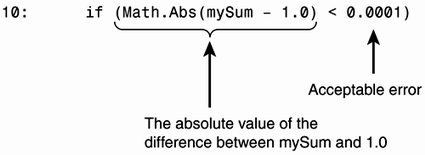Strictly speaking, you should also change line 11 to

` 11:  Console.WriteLine("mySum is close to 1.0"); `

The output is now changed to

` mySum is close to 1.0 mySum holds the value 1.0000000149011612 `
##### Adding or Subtracting Floating-Point Numbers with Different Magnitudes

Consider a variable of type float with the value 1234567000. As we have seen before, the last three zeros are mere placeholders. So, if you attempted to add or subtract a number with a very different magnitude, such as 5, it would not be registered by the variable in this case. Listing 6.11 illustrates this point. The calculation 1234567000 5 is, by standard arithmetic, equal to 1234566995, which differs from our incorrect result in the sample output for Listing 6.11.

##### Listing 6.11 Source Code for DifferentMagnitudes.cs
`01: using System; 02: 03: class DifferentMagnitudes 04: { 05:     public static void Main() 06:     { 07:         float distance = 1234567000f; 08: 09:         distance = distance - 5f; 10:         Console.WriteLine("New distance: " + distance); 11:     } 12: } New distance: 1.234567E+09 `

Obviously our operation in line 9 of the source code in Listing 6.11 is useless.

TipAdditions and subtractions involving floating-point values should not involve numbers of very different magnitudes.

#### The decimal Type

In many ways, the decimal type is similar to the floating-point types in that it allows us to represent numbers with fractions and utilizes the idea of base value, significant digits, and scalar value. However, it is significantly more precise, has a smaller range, and takes up much more memory. Thus, the decimal type is useful for calculations in need of extreme accuracy.

A Decimal Type is Not a Floating-Point TypeEven though decimal type values are used to represent fractions like floating-point types, they are not considered to be part of these types. The decimal type does not support positive/negative zeros, positive/negative infinites, or NaN as the floating-point types. Instead, values between 1.0E 28 and 1.0E 28 will all simply be zero, and values out of range will generate overflow/underflow exceptions. For decimal overflow/underflow operations, it is not possible to switch the compiler check on or off with the /checked compiler switch or the checked or unchecked operators/statements, as is the case for overflowing/underflowing integers.

The following Note lists the characteristics of the decimal type.

The decimal Type in a NutshellVariables of type decimal can represent values from approximately 7.9E28 to 7.9E28. They can get as close to zero (without holding zero itself) as 1.0E 28 or 1.0E 28. Values are represented with a staggering 28 significant digits. A decimal occupies 128 bits (16 bytes) of memory.

The decimal type solves the problem encountered on Listing 6.11 where the inaccuracy of 0.2f was revealed. In fact, decimal types can represent numbers such as 0.1 and 0.2 with 100% accuracy.

Use Integer Types Where You CanFloating-point and decimal numbers let you represent numbers with fractions and with a greater range than integers, but this comes with a price increased memory requirements and slower performance.

##### Writing Numbers of Type decimal

As stated previously, any number written with a decimal point or with the e-notation is of type double by default. Thus, if we want a literal to be of type decimal, we need to specify this explicitly by appending the number with an m (or M). As you will see shortly, integer values are implicitly converted to type decimal if required. Consequently, integer literals, such as 10, 756, and 963, need not carry the m suffix.

#### Floating-Point, decimal, and Integer Value Compatibilities

The previous sections introduced the complete range of predefined types that enable you to represent numeric values in a program. We have already looked at the compatibilities between each of the integer types. Next, I have extended this discussion to floating-point and decimal types and linked these with the integer types.

• float and double compatibilities Any value of type float can be represented by a value of type double; consequently, float type values are implicitly converted to values of type double.

Implicit conversions from double to float are not possible.

• Floating-points and decimal compatibilities The floating-point types have larger ranges than the decimal type. This might produce overflow/underflow exceptions during conversions from floating-point values to decimal values. Consequently, no implicit conversions are provided from any of the floating-point types to the decimal type.

On the other hand, the decimal type has a higher number of significant digits (higher precision) than any of the floating-point types, ruling out any implicit conversions from the decimal type to the floating-point types.

• Integers and floating-points. Integers and decimals The compiler will implicitly convert any of the integer types to both of the two floating-point types and the decimal type, despite the fact that conversions from int, uint, or long to float and from long to double could incur a loss of precision. However, because the range of float and double is far broader than any of the integer types, there is no loss of magnitude.

Based on this information and Figure 6.12 (presenting the integer type compatibilities), it is possible to illustrate how all the types discussed so far in this chapter relate in terms of possible implicit conversion paths. This is done in Figure 6.17. Please note that even though the char type has not been discussed yet, it is included in the figure for completeness. The char type will be discussed in Chapter 7 "Types Part II: Operators, Enumerators, and Strings."

##### Figure 6.17. Implicit conversion paths for the numeric types.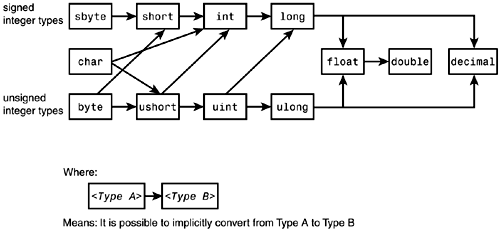#### Explicit Type Conversions

Apart from the implicit conversions that are happily and automatically performed by the compiler, C# also lets you force the compiler to perform type conversions by moving against the flow of the arrows shown in Figure 6.17. This requires a type cast explicitly written in the C# source.

More specifically, a cast operator consists of two parentheses enclosing a type (<Type>). It must be placed in front of the value you want to cast. For this reason, type casts are also termed explicit conversions. Let's illustrate the type cast with the following code snippet: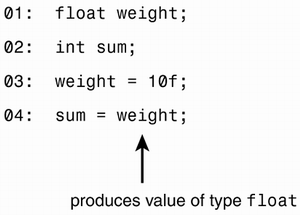Line 4 contains an invalid statement because we attempt to let the compiler perform an implicit conversion against the implicit conversion path, by assigning a variable of type float to a variable of type int. However, if the programmer is confident that in any execution of this program, the value of weight can be represented by sum of type int or, if this is not the case, the incurred data loss will not be harmful, he or she might want to enforce an explicit type conversion in line 4. This is done by placing the type cast operator (int) in front of weight in line 4, which then looks like the following: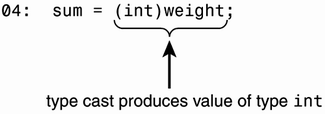Line 4 now contains the expression (int)weight, which is called a type cast.

NoteThe type cast (int)weight does not change anything about weight. Its type and its value remain untouched. (int)weight merely produces a value of type int instead of a value of type float.

If the value of weight is 15.0, the cast produces 15. If the value of weight is 15.98, the cast also produces the value 15; the 0.98 has been lost on the way to the variable sum. Note here that 15.98 has not been rounded off; instead, the fraction .98 has been removed. In computer language, this is called truncating.

## Syntax Box 6.4 The Type Cast

` Type_cast::=        (<Type>) <Expression> `

Examples

` (ushort) 6046 (decimal)((number1 + number2) / 2) Where number1 and number2 arbitrarily could be chosen to bevariables of type double. `

#### Constants: Symbolic Names for Literals

Often, a source program will make use of unchanging numbers, such as 3.141592 (Pi), 186,000 (the approximate speed of light in miles/seconds), perhaps the maximum speed of an elevator or, other values more specific to your program, that you might expect not to change during the lifetime of a program. C# allows you to declare names for the literals or other expressions in your program representing such constants and allows you to use the name instead of writing the actual value. For example, instead of writing,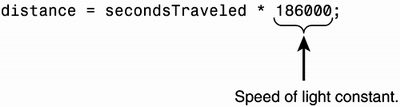you could give 186000 the name SpeedOfLight and write the same statement as follows:

` distance = secondsTraveled * SpeedOfLight; `

SpeedOfLight looks very similar to a variable in that it has a name and a value. In fact, we could have declared it to be a straightforward variable with the following line:

` int SpeedOfLight = 186000; `

This would allow us to use SpeedOfLight in our distance calculation. However, there is a problem attached to using a variable here. We might accidentally change the value SpeedOfLight somewhere else in our program. To solve this problem, we can specify the value of SpeedOfLight to remain unchanged by using the const keyword.

Line 7 of Listing 6.12 demonstrates the use of const by declaring the name MassOfElectron (line 7) to represent the constant value 9.0E-28 (mass of electron in grams). MassOfElectron is applied in line 16 to calculate the total mass of a given number of electrons.

##### Listing 6.12 Source Code for MassCalculator.cs
`01: using System; 02: 03: // Calculates the mass of a given number of electrons 04: 05: class MassCalculator 06: { 07:     const decimal MassOfElectron = 9.0E-28m; 08: 09:     public static void Main() 10:     { 11:         decimal totalMass; 12:         int electronAmount; 13: 14:         Console.WriteLine("Enter number of electrons"); 15:         electronAmount = Convert.ToInt32(Console.ReadLine()); 16:         totalMass = MassOfElectron * electronAmount; 17:         Console.WriteLine("Mass of " + electronAmount + 18:             " electrons: " + totalMass + " grams"); 19:     } 20: } Enter number of electrons 2000<enter> Mass of 2000 electrons: 1.8E-24 grams `

A name that represents a constant value is called a constant.

## Syntax Box 6.5 Declaring a Constant

` Constant_declaration_statement ::= [public | private] const <Type> <Constant_Identifier> = <Constant_expression>;`

Notes:

The <Constant_expression> here can consist of just one literal, as in the following: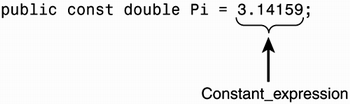It can also consist of a mixture of literals, other constants from the source code, and operators, as long as the expression can be calculated at compile time.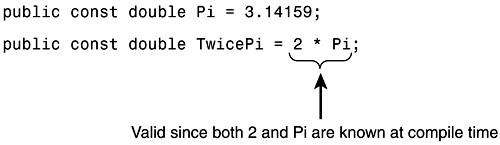The constant declaration can be placed in a class definition, making the constant a class member. Constants here are always static and so can only be accessed by other objects outside the class by using the classname (followed by the dot operator and the name of the constant) and not the instantiated object names. Listing 6.13 demonstrates this point.

##### Listing 6.13 Source Code for ConstantMass.cs
`01: using System; 02:  // Calculates the mass of 100 electrons; 03: class Constants 04: { 05:     public const decimal MassOfElectron = 9.0E-28m; 06: } 07: 08: class MassCalculator 09: { 10:     public static void Main() 11:     { 12:         decimal totalMass; 13: 14:         totalMass = 100 * Constants.MassOfElectron; 15:         Console.WriteLine(totalMass); 16:     } 17: } 9E-26 `

Listing 6.13 contains two classes, Constants and MassCalculator. The Constants class merely holds the MassOfElectron constant. The MassCalculator class needs access to this constant for its calculation of the mass of 100 electrons. Line 14 correctly contains the classname Constants followed by the dot operator (.) and the name of the constant inside the Constant class MassOfElectron, which specifies the constant Constants.MassOfElectron. On the other hand, an attempt to access the MassOfElectron constant by first instantiating the Constants class, as shown the following two lines, would be invalid.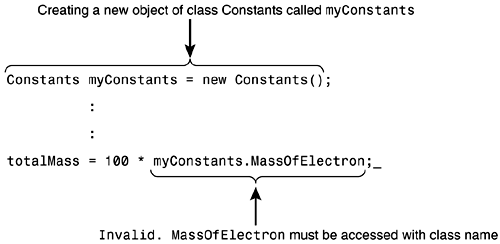NoteC# has a feature similar to constants called readonly fields. However, whereas constants must be known at compile time and will have the same value throughout the entire execution of a program, the value of a readonly field can be assigned at the time an object is created and will stay unchanged throughout the life of the object. This is a useful feature. It goes hand in hand with the constructor and initialization mechanisms surrounding object creation.

TipUse a named constant consistently throughout the code for every value in the code; don't use the named constant for some of the values and literals for the remaining. For example, if MaxSpeed represents the constant value 200 in a program, you must use MaxSpeed everywhere for this value, never the literal 200. The inconsistent use of constants is a bug-laden procedure. Imagine if one day you had to change the maximum speed (MaxSpeed) in a program with inconsistent use of named constants. You would most likely forget to change the literals and merely change the MaxSpeed declaration.

##### The Advantages of Using Constants

You have seen how to declare and use constants. But why do we need them? This section discusses a few compelling reasons for employing constants in your programs:

• Understanding the meaning of a value Instead of looking at a number like 200 somewhere in a program, MaximumSpeed gives the reader of the program a much better understanding for what the number represents. It makes the program self documenting.

• Changes only need to be made in one place Some constants can be used numerous places throughout the source code. Using literals would force you to trace all the values down one-by-one, in case you had to change the value of the constant. This is an error-prone and time-consuming process. You might overlook certain literals or inadvertently change literals with the same value but which do not represent the constant.

By having one named constant in the source, you only need to change the value in one place.

#### Formatting Numeric Values

Until now, we have been content with printing numbers using the default plain format applied when, for example, writing

` Console.WriteLine("Distance traveled: " + 10000000.432); `

which simply prints

` Distance traveled: 10000000.432 `

on the console. However, by changing the appearance of a number through embedded commas, the use of scientific notation and a limited number of decimal digits, it is possible to improve its readability and compactness when printed onscreen. Table 6.4 displays a few examples.

##### Table 6.4. Examples of Formatted Numbers
Name of variable Plain Number Formatted number with improved readability / compactness
profit 3000000000.44876 \$3,000,000,000.45
distance 7000000000000000 7.00E+015
mass 3.8783902983789877362 3.8784
length 20000000 20,000,000

In this section, we will discuss C#'s built-in features for formatting numbers when converted into strings.

##### Standard Formatting

Recall that each numeric type is based in the .NET Framework and here represented by a struct, allowing it to contain useful pre-written functionality. The ToString method is one of the methods providing this functionality. It enables us to convert any of the simple types to a string and to conveniently specify suitable formats during this conversion. Figure 6.18 shows an example utilizing the ToString method of the decimal type to convert 20000000.45965981m of type decimal to a string with commas embedded and only two decimal places shown.

##### Figure 6.18. Formatting a literal of type decimal.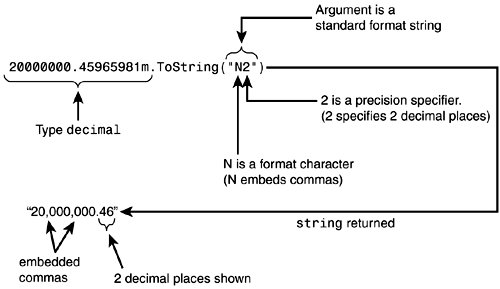The ToString method as we use it here takes one argument of type string that allows us to specify the desired string format. The argument consists of a character, called a format character, (N in this case), that indicates the format followed by an optional number called a precision specifier, (2 in this case) which has different meanings depending on the format character applied. Table 6.5 displays the different format characters available and their corresponding formats.

##### Table 6.5. Available Format Characters
Format Character Description Example
C, c

Currency.

Formatting is specific local settings. to Local settings contain information about the type of currency used and other parameters, which can vary from country to country.

2000000.456m.ToString("C") Returns: "\$2,000,000.46" (If the operating system is set to American standards.)
D, d

Integer.

Precision specifier sets the minimum number of digits. The output will be padded with leading zeros if the number of digits of the actual number is less than the precision specifier. (Note: Only available for the integer types)

45687.ToString("D8") Returns: "00045678"
E, e

E-Notation.

(Scientific) Precision specifier determines the amount of decimal digits, which defaults to 6.

345678900000.ToString("E3") Returns: "3.457E+011" 345678912000.ToString("e") Returns: "3.456789e011"
F, f

Fixed-point.

Precision specifier that indicates the number of decimal digits.

3.7667892.ToString("F3") Returns: "3.765"
G, g

General.

The most compact format of either E or F will be chosen. Precision specifier sets the maximum amount of digits number can be represented by.

65432.98765.ToString("G") Returns: "65432.98765" 65432.98765.ToString("G7") Returns: "65432.99" 65432.98765.ToString("G4") Returns: "6.543E4"
N, n

Number.

Generates a number with embedded commas. Precision specifier sets the number of decimal digits.

1000000.123m.ToString("N2") Returns: "1,000,000.12"
X, x

The precision specifier sets the minimum number of digits represented in the string. Leading zeros will be padded to the specified width.

950.ToString("x") Returns: "3b6" 950.ToString("X6") Returns: "0003B6"

Notes:

The format character can be specified either in uppercase or lowercase. However, it only makes a difference in the case of E (e-notation) and X (hexadecimal). E specifies E to be used as in "3.0E+010"; e specifies e to be used as in "3.0e+010".

X specifies uppercase letters to be used to represent the hexadecimal value as in "FFA5". x specifies lowercase letters as in "ffa5".

The ToString method provides us with powerful means to format numeric values. However, its use becomes somewhat longwinded and cumbersome if we write several formatted numbers embedded in a string. The following lines illustrate how unclear the call to WriteLine becomes and how difficult it is to keep track of which part is static text and which part is a formatted number:

` Console.WriteLine("The length is: " + 10000000.4324.ToString("N2") + "  The width is: " +65476356278.098746.ToString("N2") + "  The height is: " +4532554432.45684.ToString("N2")); `

Providing the following output:

` The length is: 10,000,000.43  The width is: 65,476,356,278.10 The height is 4,532,554,432.46 `

The statement would be much clearer if we could somehow separate the static text and the numbers and just indicate, with small discreet specifiers, the position and format of each number. C# provides an elegant solution to this problem.

If we disregard the formatting part for a moment and decide to let {<N>} be such a specifier, where <N> refers to the position of a number in a list of numbers positioned after the static text in the call to WriteLine, we can write the previous lines as follows:

` Console.WriteLine("The length is: {0} The width is: {1} The height is: {2}",          10000000.4324, 65476356278.098746, 4532554432.45684); `

where {0} refers to the first value (100000000.4324) in the list of numbers after the string; {1} refers to the second value (65476356278.098746), and {2} to the third value. Certainly, this has provided us with a much clearer statement.

Because the yet to be explored string class together with the WriteLine method provides these features, I will end this part of the story here. When we look at strings in Chapter 7, I will finish the story and discuss how you can combine format characters and precision specifiers with the WriteLine method to not only specify where a certain number is positioned but also how it is formatted.

#### The bool Type: A Brief Encounter

The bool type (see last row of Table 6.2) is named after George Boole (1815 1864) an English mathematician who devised a formal way of representing and working with Boolean expressions, called Boolean algebra.

Just like a value of type short can hold any value between 32768 and 32767, a value of type bool can hold one of the literal values true and false, which are keywords in C#.

Whereas conventional algebra of arithmetic expresses the rules abided by addition, multiplication, and negation of numbers, Boolean algebra describes the rules obeyed by the logic operators "and," "or," and "not" when combined with the two values true and false.

Boolean algebra, together with the bool type, form the foundation of a powerful system of logic enabling you to think and reason effectively about the design of computer programs. Consequently, it is impossible to create C# programs of much substance without applying the bool type together with at least some of the rules found in Boolean algebra.

An expression that returns either the value true or false is termed a Boolean expression.

The following is an example of a Boolean expression

` (length > 100) `

returns true if the variable length is greater than 100 and false if length is smaller than or equal to 100.

true and false represent our intuitive understanding of the concepts true and false and allow us to specify the correctness of a claim (represented by a variable) by simply declaring a bool variable

` bool isFinished; `

and assigning it the literal value true (or false) as in the following:

` isFinished = true; `

This also allows us to assign any value from an expression that will return either true or false. For example, we could declare the bool variable distanceIsGreaterThanTen

` bool distanceIsGreaterThanTen; `

and assign it the value of the Boolean expression (distance > 10) as in the following:

` distanceIsGreaterThanTen = (distance > 10); `

It also allows us to let it be part of the condition of an if statement such as the following:

` if(distanceIsGreaterThanTen)       Console.WriteLine("Distance is greater than ten"); `

which only prints "Distance is greater than ten" if distanceIsGreaterThanTen contains the value true.

Statements controlling the flow of a program, such as if statements and do-while loops, go hand in hand with the bool type and Boolean algebra. This section is just a brief introduction to the comprehensive treatment of the subject found in Chapter 8, "Flow of Control Part 1: Branching Statements And Related Concepts."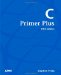C Primer Plus (5th Edition)
ISBN: 0672326965
EAN: 2147483647
Year: 2000
Pages: 286
Authors: Stephen Prata